Minima/ maxima (statement questions) - Geometry questions

Chapter 6 Class 12 Application of Derivatives
Concept wise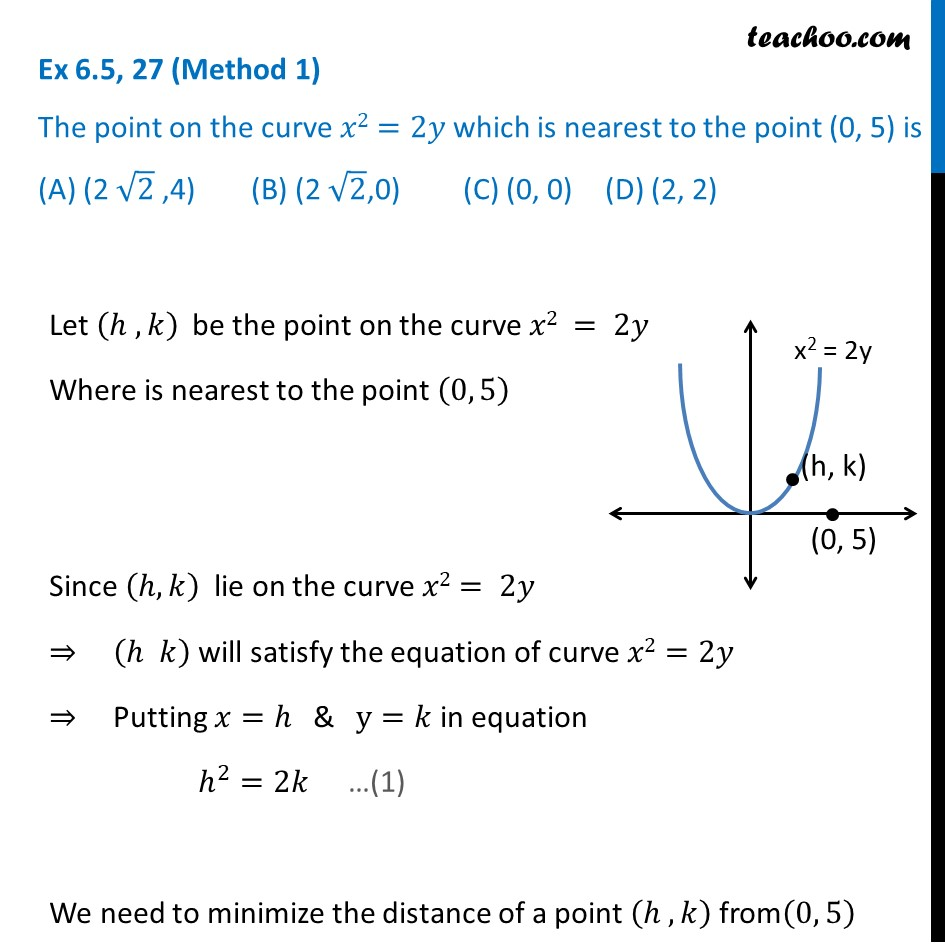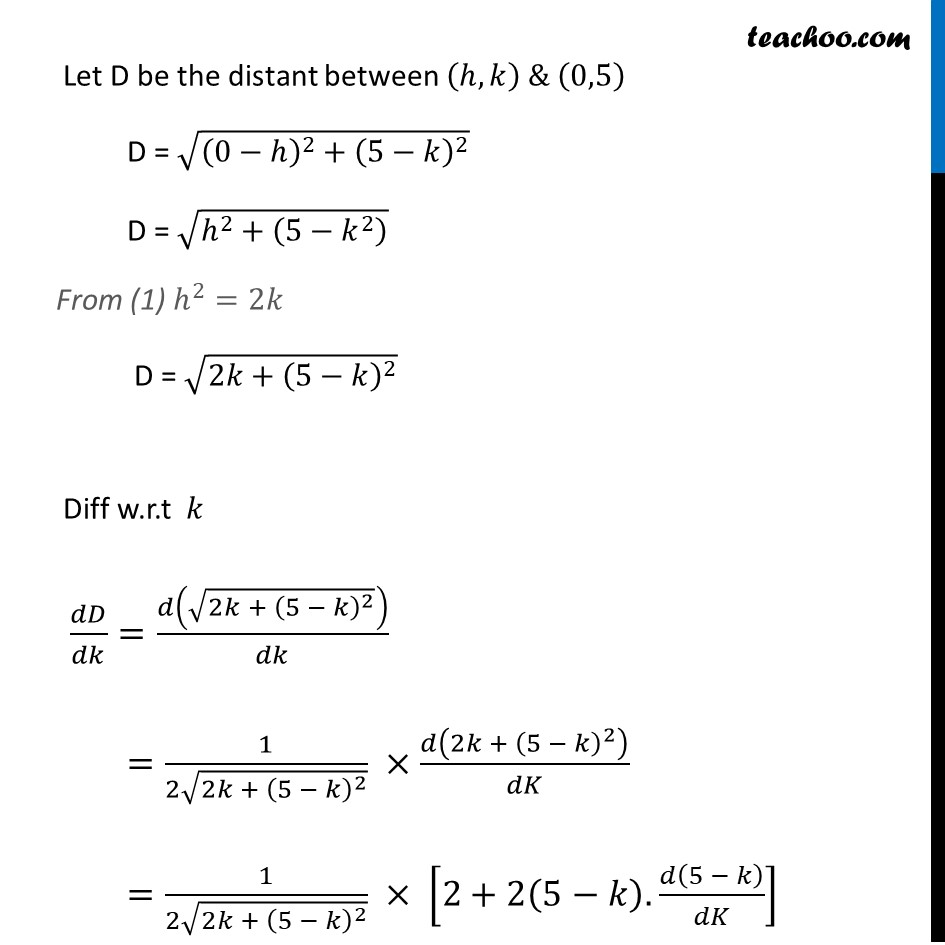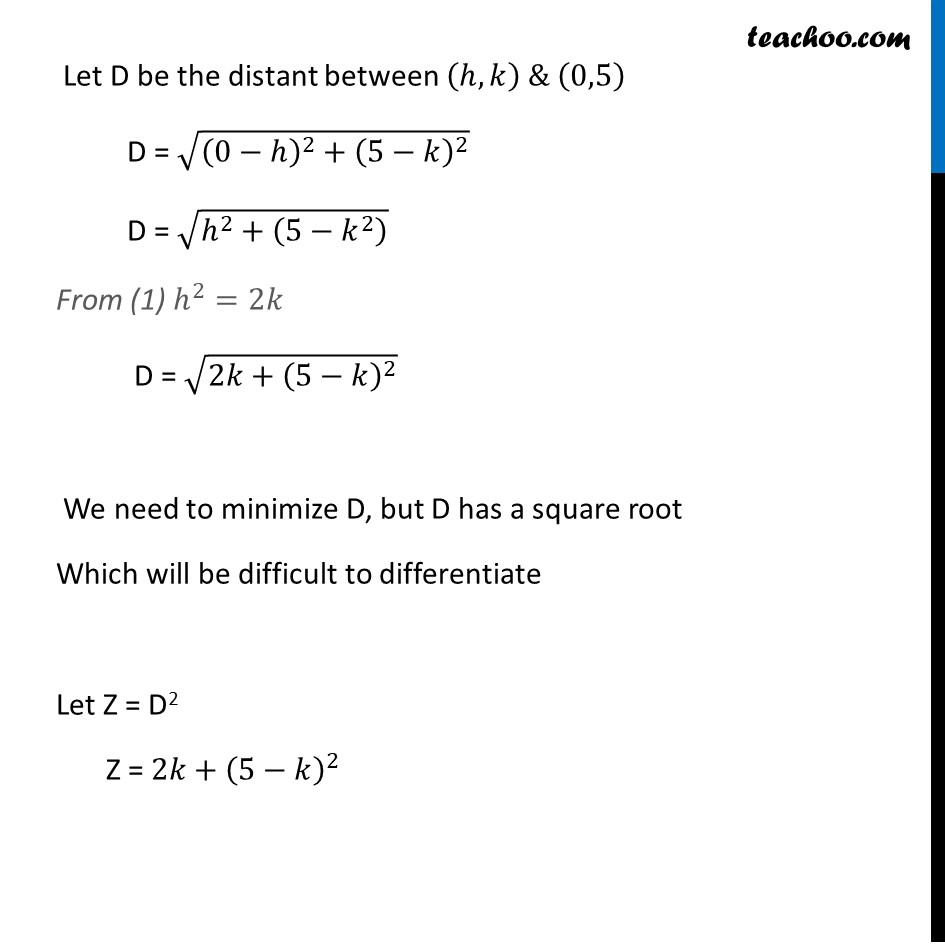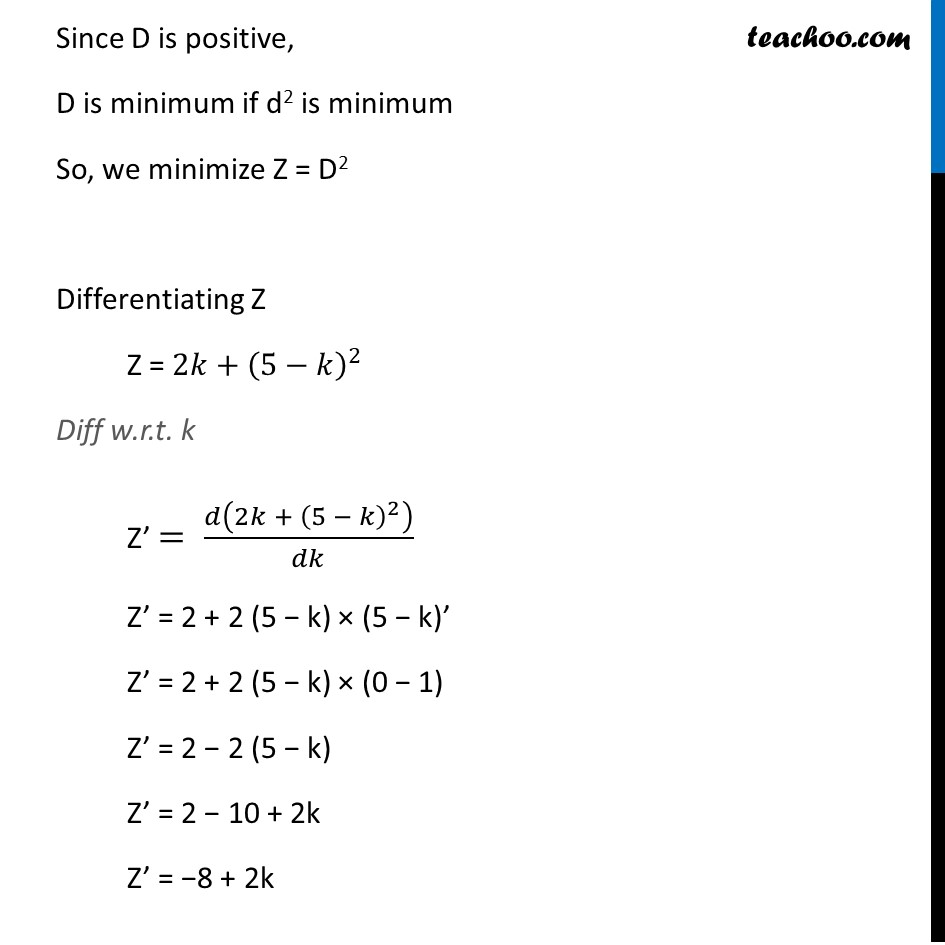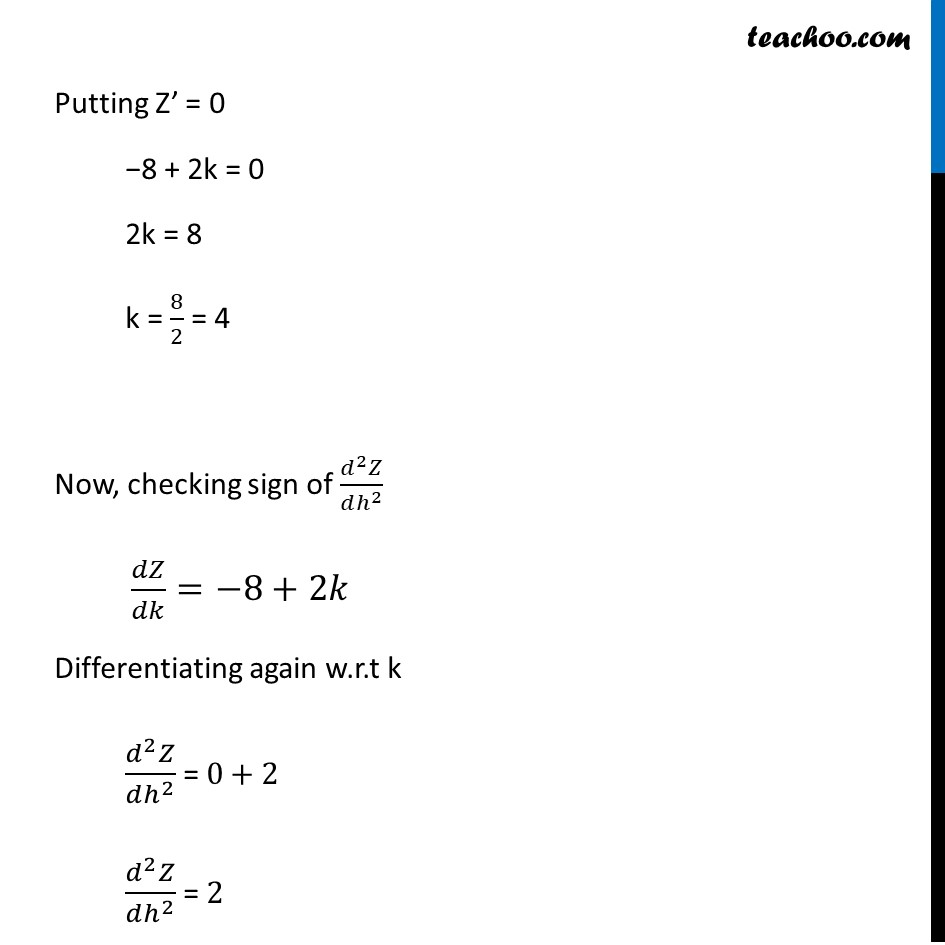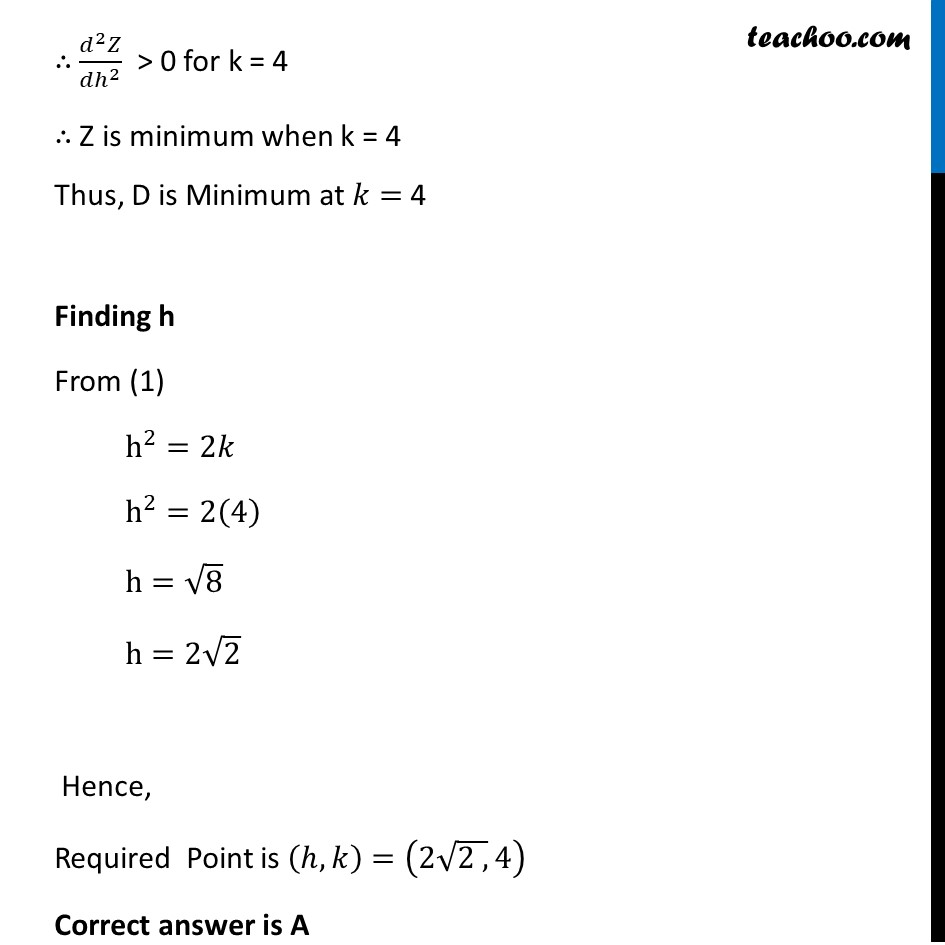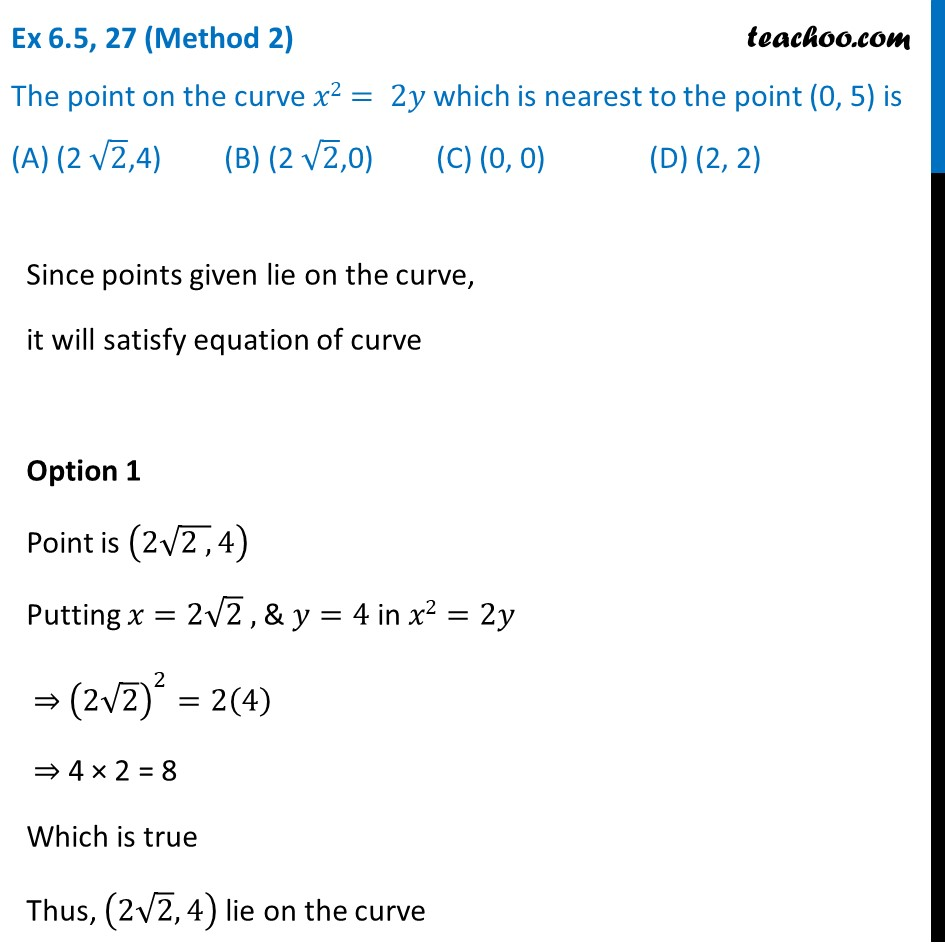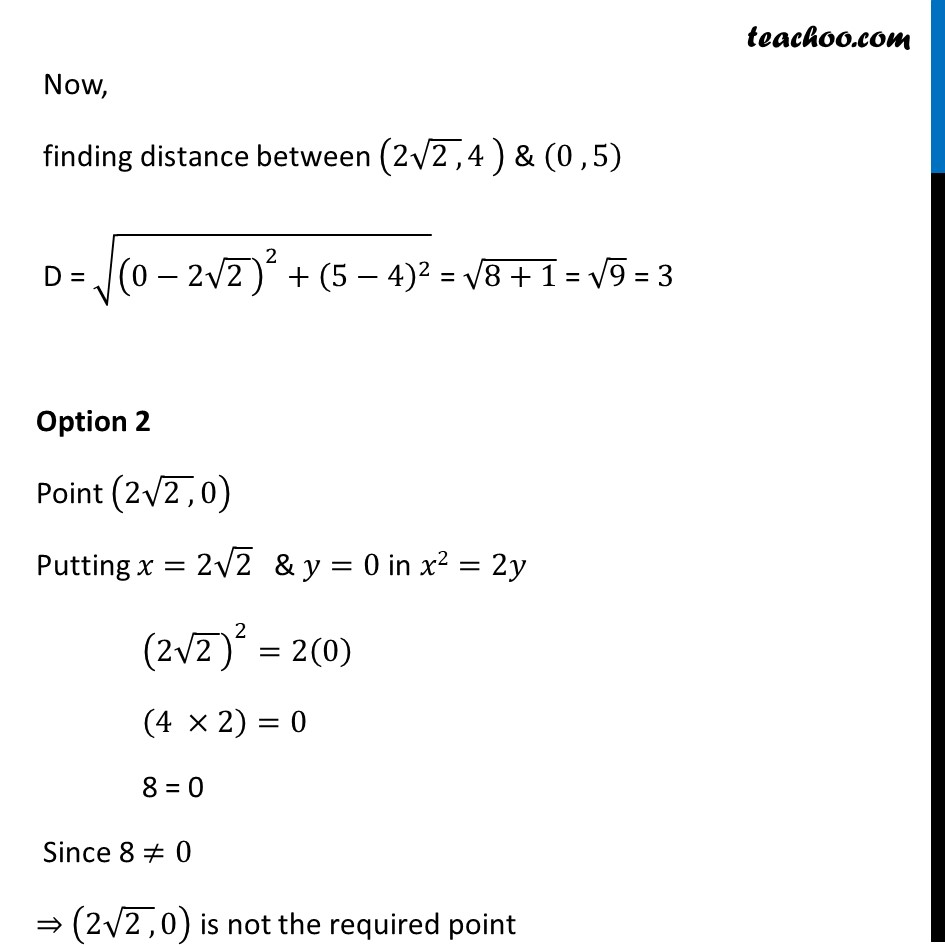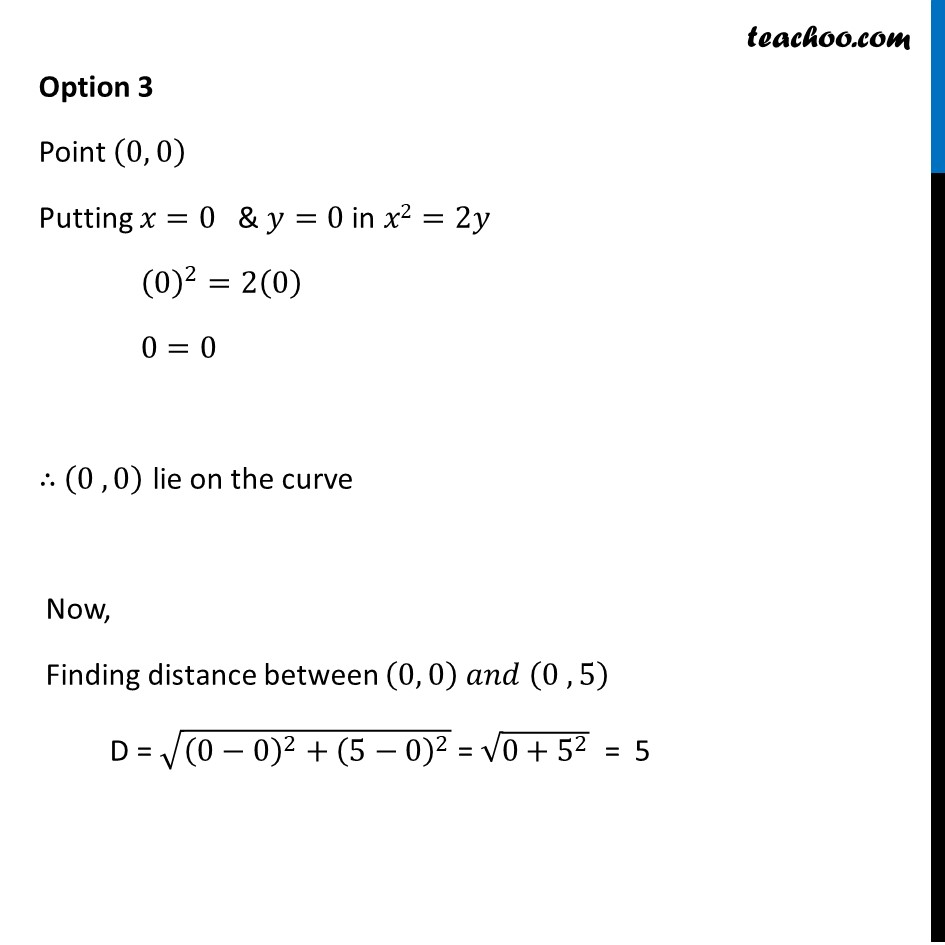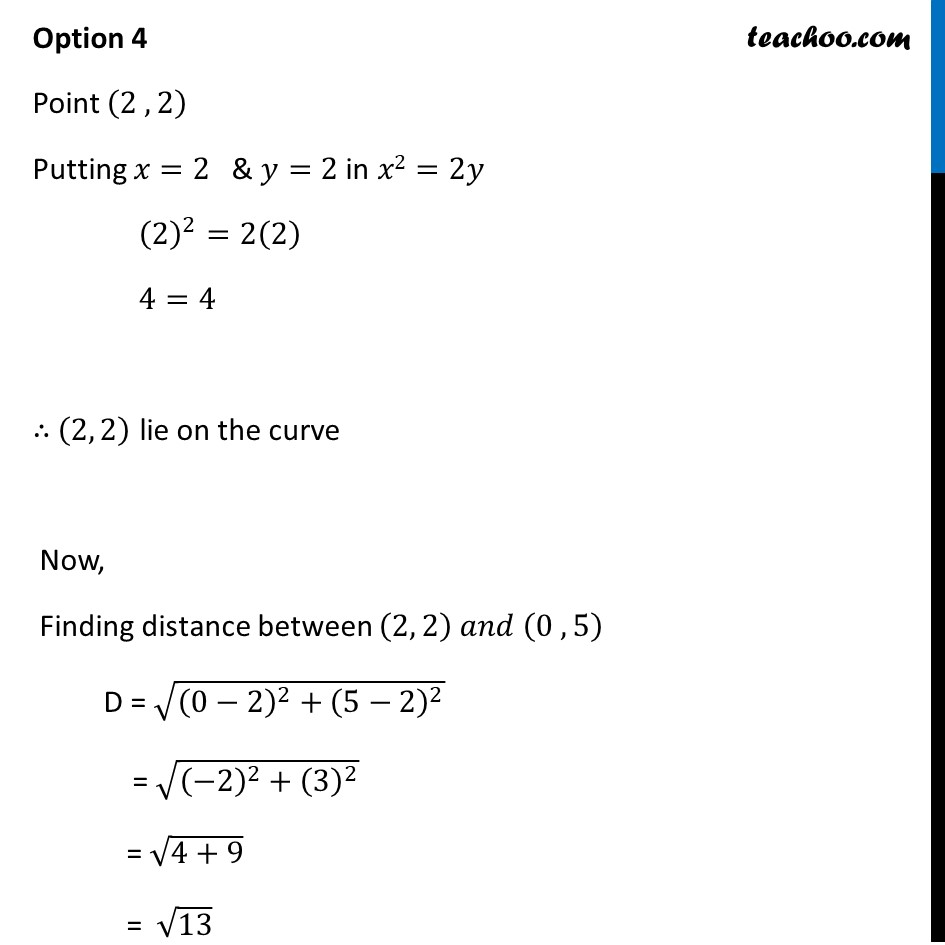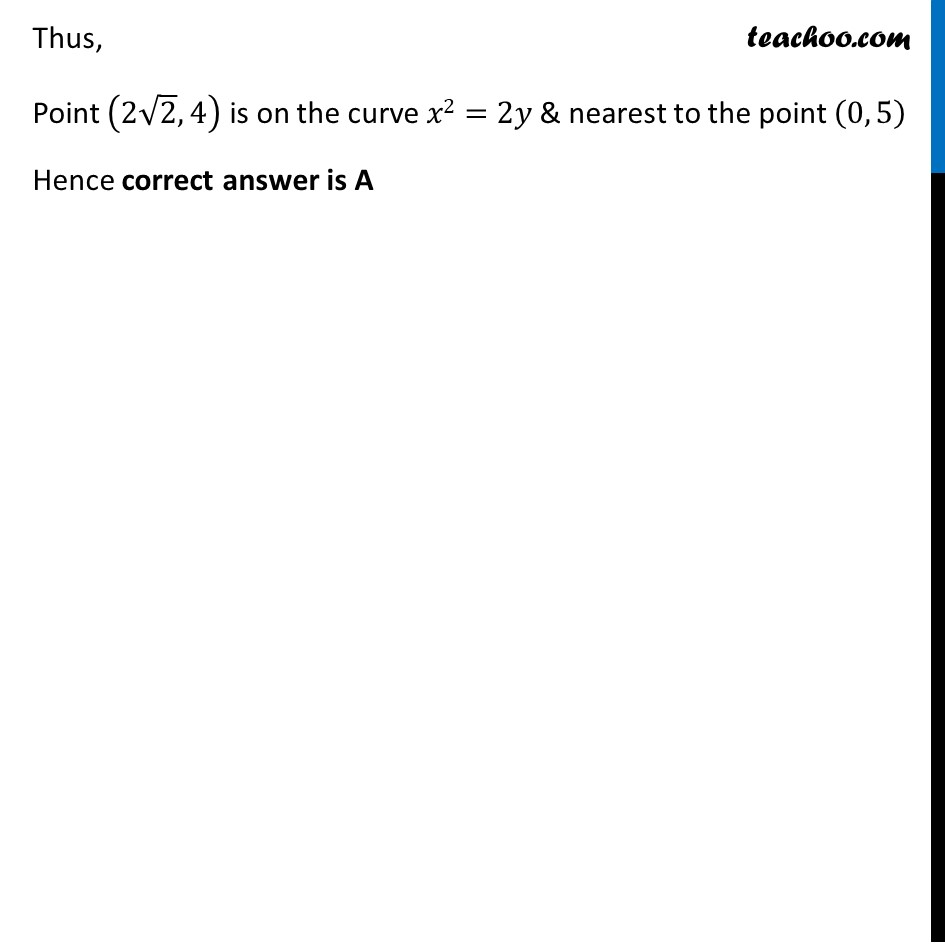Introducing your new favourite teacher - Teachoo Black, at only ₹83 per month

### Transcript

Ex 6.5, 27 (Method 1) The point on the curve 𝑥2=2𝑦 which is nearest to the point (0, 5) is (A) (2 √2 ,4) (B) (2 √2,0) (C) (0, 0) (D) (2, 2) Let (ℎ , 𝑘) be the point on the curve 𝑥2 = 2𝑦 Where is nearest to the point (0, 5) Since (ℎ, 𝑘) lie on the curve 𝑥2= 2𝑦 ⇒ (ℎ 𝑘) will satisfy the equation of curve 𝑥2=2𝑦 ⇒ Putting 𝑥=ℎ & y=𝑘 in equation ℎ^2=2𝑘 We need to minimize the distance of a point (ℎ ,𝑘) from(0, 5) Let D be the distant between (ℎ,𝑘) & (0,5) D = √((0−ℎ)^2+(5−𝑘)^2 ) D = √(ℎ^2+(5−𝑘^2 ) ) From (1) ℎ^2=2𝑘 D = √(2𝑘+(5−𝑘)^2 ) Diff w.r.t 𝑘 𝑑𝐷/𝑑𝑘=𝑑(√(2𝑘 + (5 − 𝑘)^2 ))/𝑑𝑘 =1/(2√(2𝑘 + (5 − 𝑘)^2 )) ×𝑑(2𝑘 + (5 − 𝑘)^2 )/𝑑𝐾 =1/(2√(2𝑘 + (5 − 𝑘)^2 )) × [2+2(5−𝑘).𝑑(5 − 𝑘)/𝑑𝐾] Let D be the distant between (ℎ,𝑘) & (0,5) D = √((0−ℎ)^2+(5−𝑘)^2 ) D = √(ℎ^2+(5−𝑘^2 ) ) From (1) ℎ^2=2𝑘 D = √(2𝑘+(5−𝑘)^2 ) We need to minimize D, but D has a square root Which will be difficult to differentiate Let Z = D2 Z = 2𝑘+(5−𝑘)^2 Since D is positive, D is minimum if d2 is minimum So, we minimize Z = D2 Differentiating Z Z = 2𝑘+(5−𝑘)^2 Diff w.r.t. k Z’ = 𝑑(2𝑘 + (5 − 𝑘)^2 )/𝑑𝑘 Z’ = 2 + 2 (5 − k) × (5 − k)’ Z’ = 2 + 2 (5 − k) × (0 − 1) Z’ = 2 − 2 (5 − k) Z’ = 2 − 10 + 2k Z’ = −8 + 2k Putting Z’ = 0 −8 + 2k = 0 2k = 8 k = 8/2 = 4 Now, checking sign of (𝑑^2 𝑍)/(𝑑ℎ^2 ) " " 𝑑𝑍/𝑑𝑘=−8+2𝑘 Differentiating again w.r.t k (𝑑^2 𝑍)/(𝑑ℎ^2 ) = 0+2 (𝑑^2 𝑍)/(𝑑ℎ^2 ) = 2 ∴ (𝑑^2 𝑍)/(𝑑ℎ^2 ) > 0 for k = 4 ∴ Z is minimum when k = 4 Thus, D is Minimum at 𝑘= 4 Finding h From (1) h^2=2𝑘 h^2=2(4) h=√8 h=2√2 Hence, Required Point is (ℎ,𝑘)=(2√(2 ,) 4) Correct answer is A Ex 6.5, 27 (Method 2) The point on the curve 𝑥2= 2𝑦 which is nearest to the point (0, 5) is (A) (2 √2,4) (B) (2 √2,0) (C) (0, 0) (D) (2, 2)Since points given lie on the curve, it will satisfy equation of curve Option 1 Point is (2√(2 ,) 4) Putting 𝑥=2√2 , & 𝑦=4 in 𝑥2=2𝑦 ⇒ (2√2)^2=2(4) ⇒ 4 × 2 = 8 Which is true Thus, (2√2,4) lie on the curve Now, finding distance between (2√(2 ,) 4" " ) & (0 ,5) D = √((0−2√(2 ))^2+(5−4)^2 ) = √(8+1) = √9 = 3 Option 2 Point (2√(2 ,) 0) Putting 𝑥=2√2 & 𝑦=0 in 𝑥2=2𝑦 (2√(2 ))^2=2(0) (4 ×2)=0 8 = 0 Since 8 ≠0 ⇒ (2√(2 ,) 0) is not the required point Option 3 Point (0, 0) Putting 𝑥=0 & 𝑦=0 in 𝑥2=2𝑦 (0)^2=2(0) 0=0 ∴ (0 , 0) lie on the curve Now, Finding distance between (0, 0) 𝑎𝑛𝑑 (0 , 5) D = √((0−0)^2+(5−0)^2 ) = √(0+5^2 ) = 5 Option 4 Point (2 ,2) Putting 𝑥=2 & 𝑦=2 in 𝑥2=2𝑦 (2)^2=2(2) 4=4 ∴ (2, 2) lie on the curve Now, Finding distance between (2, 2) 𝑎𝑛𝑑 (0 , 5) D = √((0−2)^2+(5−2)^2 ) = √((−2)^2+(3)^2 ) = √(4+9) = √13 Thus, Point (2√2,4) is on the curve 𝑥2=2𝑦 & nearest to the point (0, 5) Hence correct answer is A